This article will take you through the fundamentals of python and one of the data science python library i.e. Numpy that is the Library  to get the data in the required format in order to draw out conclusions in terms of bring Intelligence out of the data.

## Quick Basics of python

#### 1. What is an interpreter?

An interpreter is a program that reads and executes your source code, pre-compiled codes, scripts. It also manages your modules/packages of the programming language on your machine in different way.

#### 2. Difference between the virtual environment and the existing interpreter?

The difference is that if you use a virtual environment for your project and add/remove packages then it will only affect the virtual environment. If you use an existing interpreter then all changes will affect the system-wide interpreter and these changes will be available in all the projects that use that interpreter.

#### 3. What is a pip?

Pip is a standard package management system used to install and manage the software packages written in python.

4. What are the various commands of pip?

Ans: Below are the various commands of pip to be run in the command prompt/terminal

• List all the packages-> pip list
• Install package -> pip install package Name; an example is:  pip install Numpy
• Uninstall package -> pip uninstall package Name
• Search a package -> pip search package Name

#### 5. What are variables?

Variables are used to store information to be referenced and manipulated in a program. In python, we don’t need to explicitly mention the datatype during declaration. A string variable cannot be manipulated by mathematical actions.

#### 6. What are the basic operations in python?

We have ‘+’ (addition) ;  ‘-‘ (subtraction) ; ‘*’ (multiplication);  ‘/’ (division); ‘%’ (modulus meaning the remainder of division) ; ‘**’ (power meaning ab i.e. a to the power of b);  ‘//’ (floor division meaning this will give the quotient of division without the decimal).

#### 7. What are string indexing and slicing?

Index is the position of each character starting from 0.

Slicing is getting the substring i.e. subset out of a string value or a word or sentence.

You slice your butter so that the chunks can be used for various purposes !! Right !! SAME IS APPLICABLE HERE AS WELL !!

Suppose we have a string variable which has‘stay positive’ stored in it. The below picture shows the indexing of the elements in the string.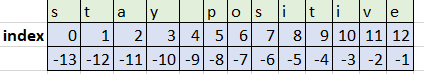Below is a code snippet having examples of indexing and slicing.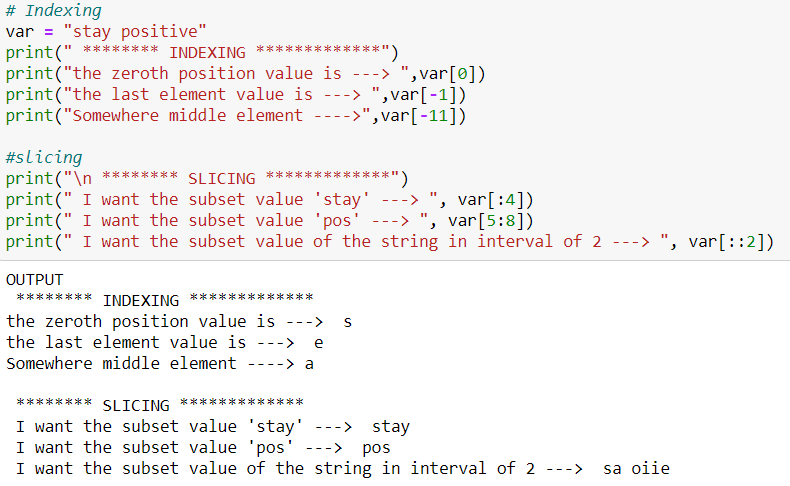#### 8. What is mutable and immutable property?

Mutability means you can change the values of an object after it is created, and the Immutable property of an object means it cannot be changed after it is created.

#### 9. What are the different data structures of python?

: Below are different types of data structures :

9. i) Lists : These are the data types that hold elements of different/same datatype together in a collection in sequential manner. These are enclosed in square brackets [ ]. Lists are mutable and indexable in nature, and also allow duplicates. There are many list methods like list.append(), list.pop(), list.reverse(),list.sort(),list.count(),list.insert(),list.remove() etc.  for performing various list operations; few of which is showed in the below code snippet.

NOTE:  Accessing and indexing elements in the list are the same as the Q7(indexing and slicing) topic explained above.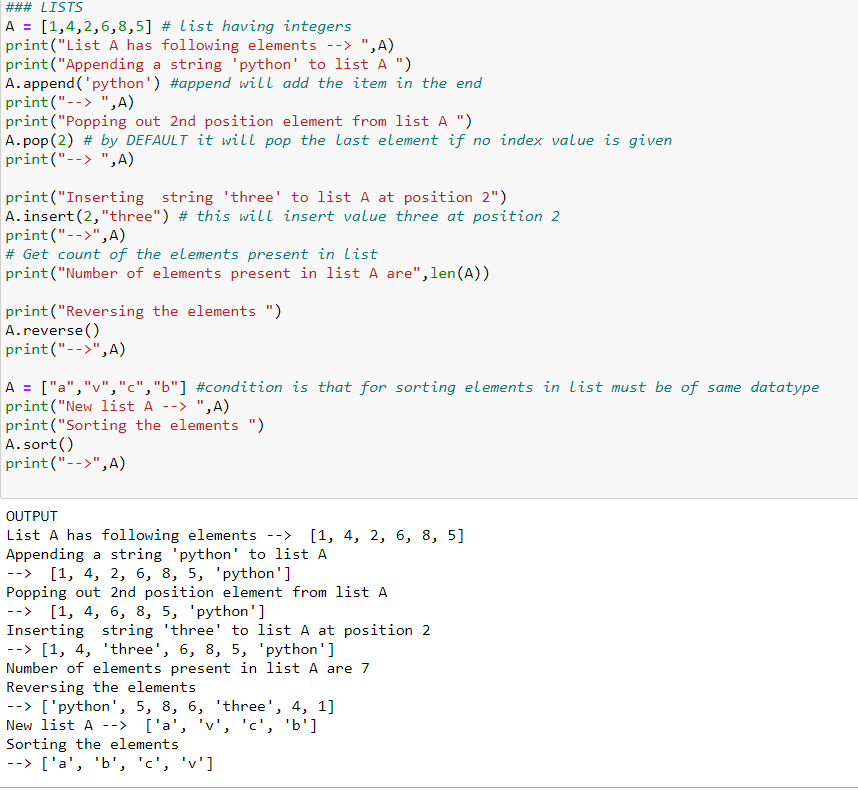9. ii) Tuples: These are similar to lists with two major differences i.e. (that is) Firstly they are enclosed within round brackets() and second they are immutable in nature.  There are two inbuilt methods that can be used on tuples index() and count(). Code snippet for same is mentioned below:

NOTE: Accessing and indexing elements in the tuples are the same as the Q7(indexing and slicing) explained above.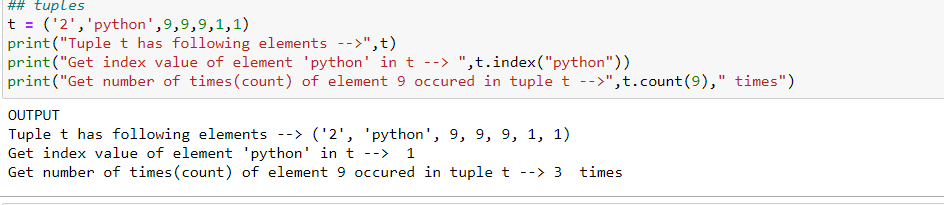9. iii) Sets: Sets are nothing but an unordered collection of data. Unordered meaning NOT IN ORDER !! Simple !! Sets are enclosed in curly braces {}. These are immutable, non-indexed. Sets do not allow duplicate elements, unlike lists or tuples that allow duplicate elements. There many set operations and methods like union(|) , intersection (&) , add(),pop(),remove() etc. Below is the code snippet for a few of them: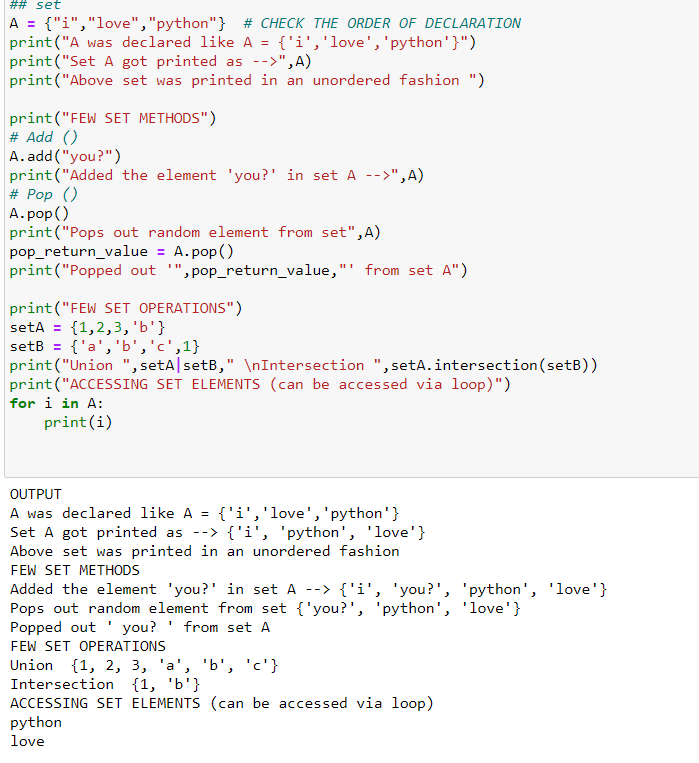9. iv) Dictionaries: Dictionaries in python is a data structure that stores the values against its keys. Basically key-value pair. It is enclosed within curly braces having key:value pair i.e. {key1:val1}

Eg-> mydict = {“classA”:30,”classB”:50,”classC”:45}

In the above example, ‘mydict’ is a dictionary that stores the number of students present in each class. So classA is key and 30 is its value.

Dictionaries do not allow duplicate keys and are mutable in nature. Below is the code snippet having dictionary examples: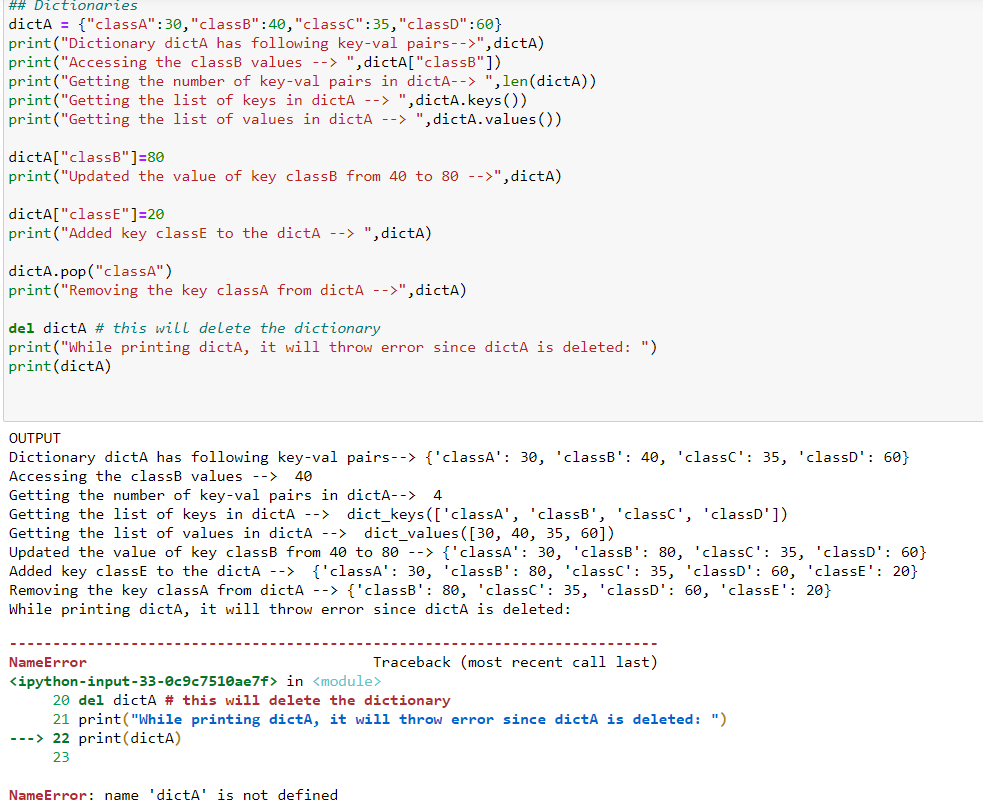There are few more functions like get(keyname) that will return the value of that key, update(),popitem(), etc. . We can also have nested dictionaries,                                                            lists value for the key i.e. key1 : [1,2,3].

What is the use of dictionary  ?

There might be scenarios wherein you will have to count the number of occurrences of an item in a list, then you can easily compute this using dictionary. Another example is using a dictionary like a lookup file wherein you might have a set of static key-value pairs to refer to. Also, dictionaries are used in backend code while building APIs. Hence with dictionaries in place, many operations like I mentioned above become easier to deal with.

10. What are the various common libraries used in Data Science?

Ans: Common libraries are :

Numpy -> Used to store data in form of an array and computing numerical operations. Numpy stands for Numerical Python

Pandas -> Used mainly for storing datasets and for data manipulation. Pandas stand for Python Data Analysis library

Matplotlib -> Used to plot the graphs, charts, histograms of data for a better understanding of data

#### 11. Why is Numpy required when we have python Lists? Since both do the same work of storing data in array form???

Ans: Absolutely, but Numpy is better since it takes less memory as compared to lists. Also, a Numpy array is faster than a list.

Now the question is HOW ??? Please follow the below code snippet showing the answer for the question of HOW  IT TAKES LESS MEMORY AND IS FASTER THAN LISTS??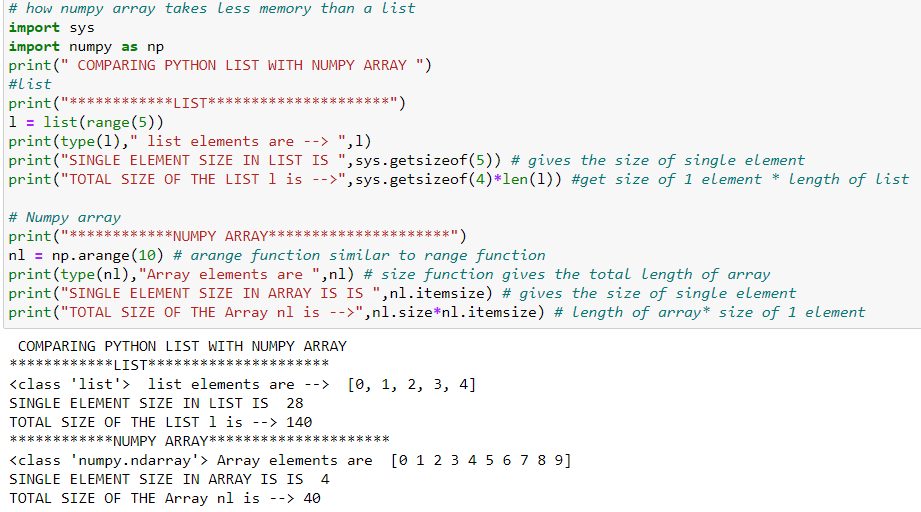In the above code, we have compared the memory used by the list and the memory used by the Numpy array. The size of a single integer element in the list takes 28 bytes whereas a Numpy array takes only 4 bytes. This is because lists are python object which requires memory for pointers as well as value, but Numpy array does not have pointers that will point to the value. Hence IT TAKES LESS MEMORY.

HOW NUMPY ARRAY  OPERATIONS ARE FASTER THAN LIST ??

Let us PROVE IT IN BELOW CODE SNIPPET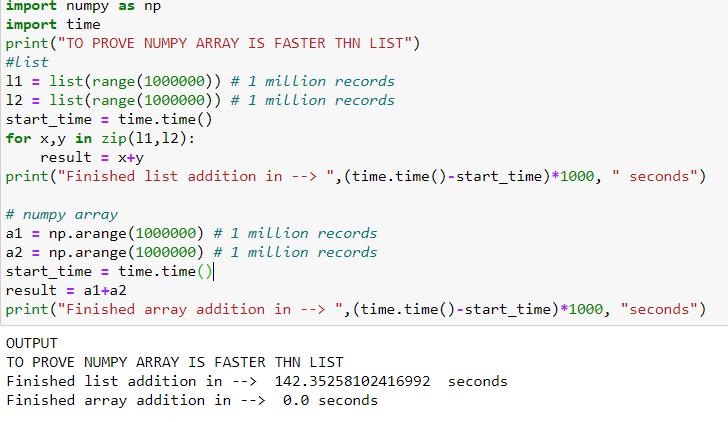In the above code, we have computed the time taken by the addition of two lists each having 1 million records that took142.3 seconds whereas when we performed the same operation with the same number of records with two arrays, the computation took 0.0 seconds !!!!! WOW!!!!

HENCE PROVED! Numpy array is much faster than a list.

In real-time, we have a huge amount of data that needs to process and analyzed so as to get useful and strategic information out of the data. Hence Numpy arrays are better than a list.

#### 12. Can we create and access the n-D(n-dimension) array using the Numpy library?

Definitely, this is one more key feature of the Numpy libraryWe can create an n-dimensional array using the array() method of Numpy by passing a list, tuple, or an array-like object.

In order to know the number of dimensions an array has, we have the “ndim” attribute of Numpy arrays.

We can also explicitly define the dimension for an array by using “ndmin” argument of the Numpy array() method.

There is a “dtype” property that will return the data type of array. Also, we can also define the data type of array by passing an argument of dtype to the array method.

Below is the code snippet for the same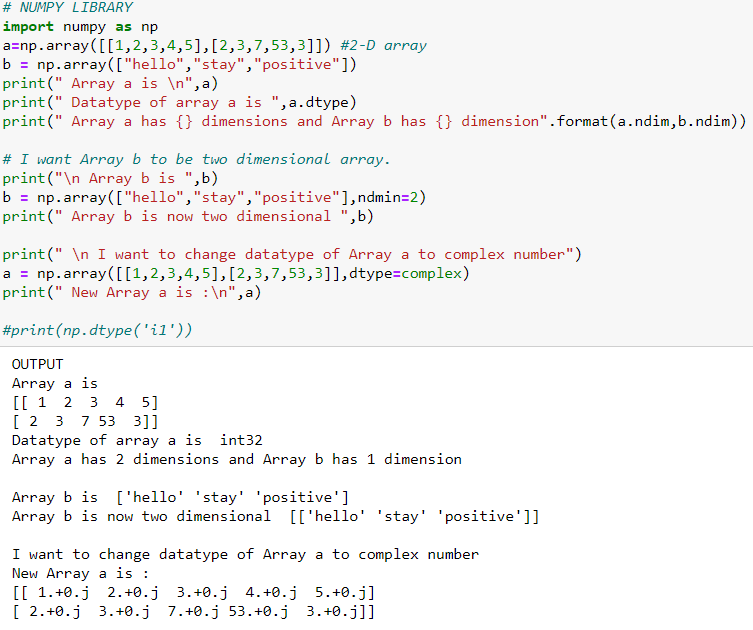#### 13. How to index, access, and  perform slicing on an n-D Numpy Array

Indexing
in Numpy array is similar to the indexing concept mentioned in the list. Below is the indexing provided for 1-D, 2-D, and 3-D arrays along with the code snippet below:

These are the positions assigned internally in the n-D array. Keeping this in mind, an n-D array can be accessed, manipulated, etc.

Please follow the below code snippets to understand how to access and slice the n-dimensional arrays.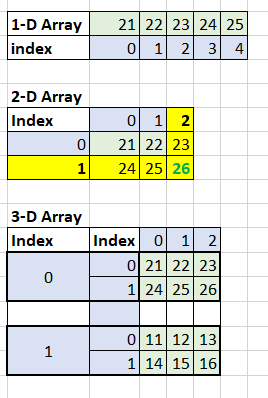The above picture represents how the indexes are represented in an n-dimensional array. Using the indexes, we can access array elements and perform slicing.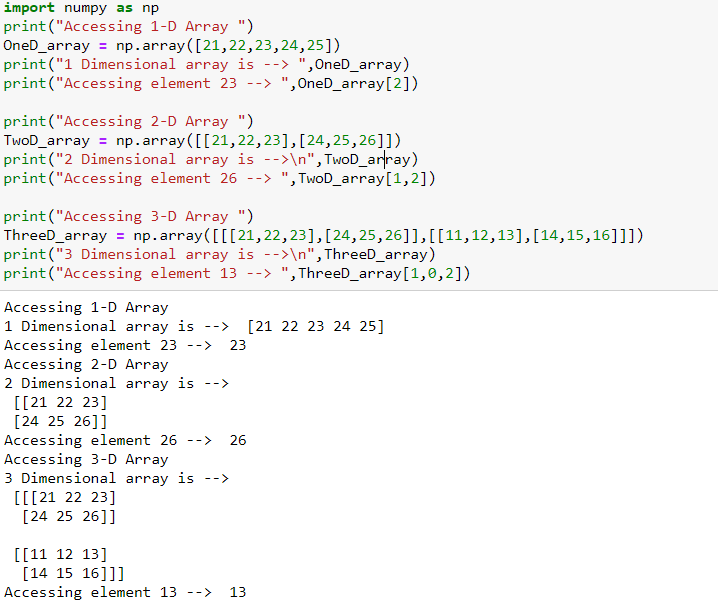SLICING:  The concept of slicing remains the same as mentioned in the above queries. The syntax for slicing is arrayName[startIndex:stopIndex:step(optional)]

Be it 1-D,2-D, or n-D, array slicing works the same.

The array examples used in the below code snippet are the same as in above eg i.e. oneD_array, TwoD_array, ThreeD_array. Please refer to the array declarations in the above code snippet.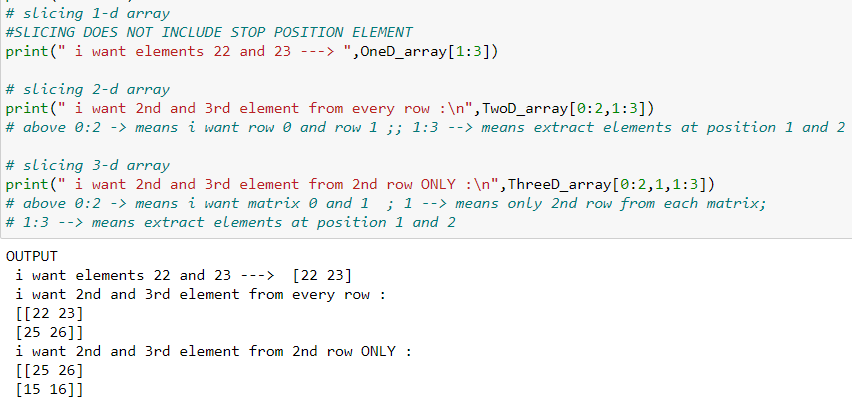#### 14. What are various methods and attributes in Numpy?

Ans: Numpy has various attributes and methods that can tell you the size, shape of the array(rows X columns), change the shape(reshape), size of each element, datatypes, and many more. Few are listed in below code snippet: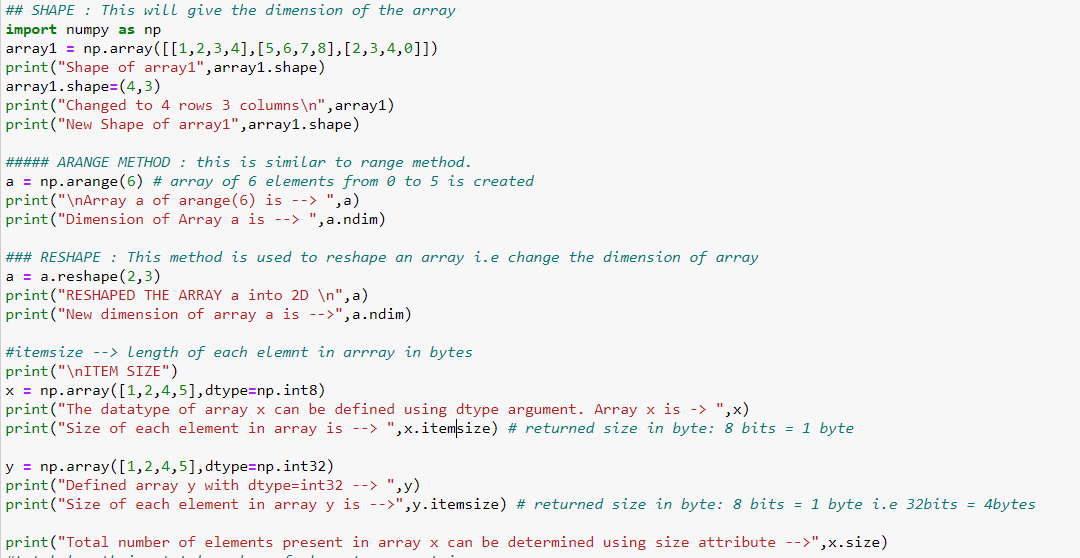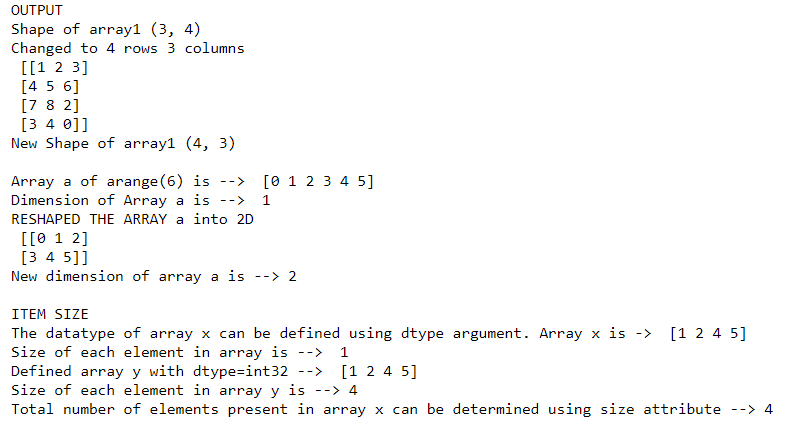We also have copy and view methods that duplicate an existing array. But these two methods do have a very major difference internally. Please find the below code snippet wherein the difference is shown in a practical way: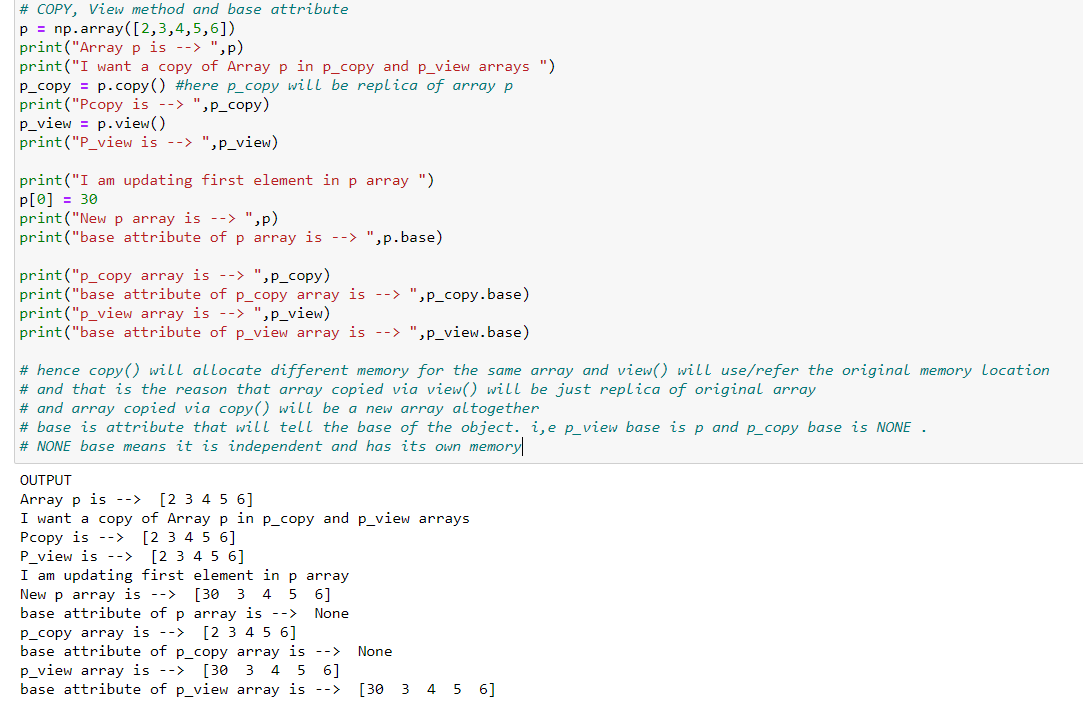Numpy also has many more methods and attributes like :

•  np.sort(array)-> This will sort the given array
• np.mean(array)-> Calculates the average of the elements present in the array
• np.zeroes((n,m))-> initializes the array with zero for n X m dimensions. A similar function we have for ones as well.
• np.linspace(start,stop,n) -> This function returns the ‘n’ numbers which are linearly spaced between start and stop values.
• array.ravel() -> ravel() function converts n-dimensional array to 1-D array.
• array.min(); array.max(); array.sum() -> As the name means getting the minimum element in the array, maximum element in the array, the summation of elements in array
• np.sqrt(array)-> This will return the square root of all the elements of array
• np.std(array)-> returns the standard deviation of the elements in the array

and Many More. You can go through all of them on the Numpy website. The ones that I have listed are the common ones that are frequently used.

ARRAY OPERATIONS :

Very easy addition, subtraction, multiplication, division can be done between two arrays. Below is the code snippet for adding and subtracting. Others can also be done in the same manner viz (a*b),(a/b)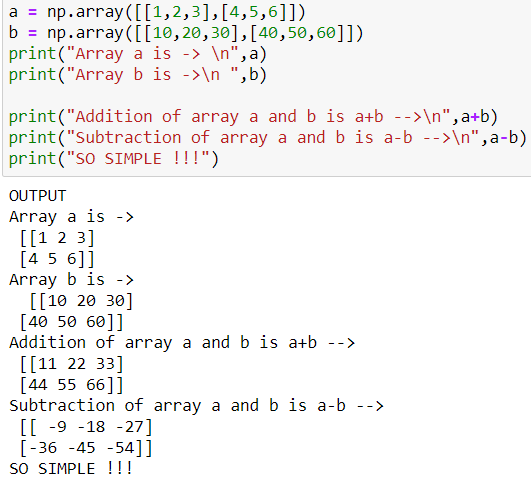#### 15. Numpy array has an Amazing Property !!  What is it ??

Ans: Let us assume we have a 2-D array where, I want to check if that every array element is greater than value 10. If yes, then replace them with True otherwise False. So in return, I will get TRUE FALSE MATRIX. Below is the code snippet: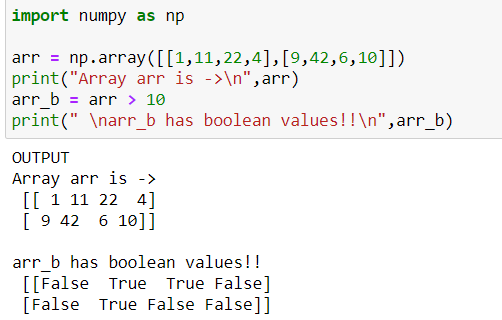Now, if I want the values of array ‘arr’ which is greater than 10 ??  This can be achieved in just a line as shown in the below code snippet: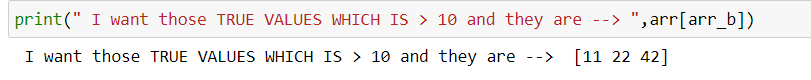Here, it extracted values from arr_b where ever it had a True value i.e. val > 10

Now we can also replace these with specific flag values like -1 or 0 or anything.

The code snippet is as follows: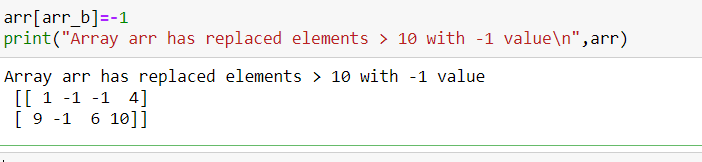So all those elements greater than 10 is replaced by -1